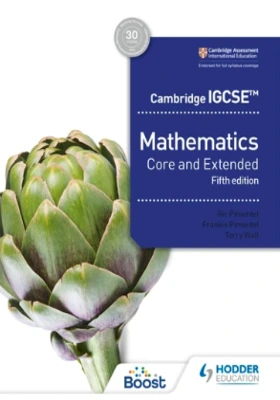Viva Group has been honoured with the Best Publisher Award 2022 by the Delhi State Booksellers & Publishers' Association.

#### Cambridge IGCSE Mathematics Core and Extended, 5/e## Cambridge IGCSE Mathematics Core and Extended, 5/e

• By: Ric Pimentel, Frankie Pimentel & Terry Wall

₹1,975.50 ₹2,195.00 Save: ₹219.50 (10%)

• Out of Stock

ISBN: 9781398373914

Bind: Paperback

Year: 2023

Pages: 576

Size: 7.5 x 9.5 Inch

Publisher: Hodder Education

Exclusive Distributors: Viva Books

Sales Territory: India, Nepal, Pakistan, Bangladesh, Sri Lanka

Description:

This title is endorsed by Cambridge Assessment International Education.

Confidently deliver and ensure full coverage of the latest Cambridge IGCSE™ Mathematics syllabuses (0580/0980) using a tried-and-tested approach to improve mathematical skills with an emphasis on problem-solving and interrogating findings in new topics.

• Put theory into practice with worked examples that show full solutions, plus plenty of exercise questions covering both Core and Extended content, all put together by an experienced team of authors.
• Encourage full understanding of mathematical principles with commentaries and additional explanations.
• Develop problem-solving skills with guidance on techniques to help complete open-ended investigations and justify reasoning for solutions.
• Check understanding with end of chapter student assessment questions to consolidate learning and test skills.
• Numerical answers to all questions are available free on hoddereducation.com/cambridgeextras.
• Worked solutions for the student assessments are available in the Cambridge IGCSE Core and Extended Mathematics Teacher’s Guide with Boost subscription.

Target Audience

IGCSE students studying maths.

Contents

Introduction • How to use this book

TOPIC 1. Number • Chapter 1. Number and language • Chapter 2. Accuracy • Chapter 3. Calculations and order • Chapter 4. Integers, fractions, decimals and percentages • Chapter 5. Further percentages • Chapter 6. Ratio and proportion • Chapter 7. Indices, standard form and surds • Chapter 8. Money and finance • Chapter 9. Time • Chapter 10. Set notation and Venn diagrams • Mathematical investigations and ICT 1

TOPIC 2. Algebra and graphs • Chapter 11. Algebraic representation and manipulation • Chapter 12 . Algebraic indices • Chapter 13. Equations and inequalities • Chapter 14. Graphing inequalities and regions • Chapter 15. Sequences • Chapter 16. Proportion • Chapter 17. Graphs in practical situations •
Chapter 18. Graphs of functions • Chapter 19. Differentiation and the gradient function • Chapter 20. Functions • Mathematical investigations and ICT 2

TOPIC 3. Coordinate geometry • Chapter 21. Straight line graphs • Mathematical investigations and ICT 3

TOPIC 4. Geometry • Chapter 22. Geometrical vocabulary and construction • Chapter 23. Similarity and congruence • Chapter 24 . Symmetry • Chapter 25. Angle properties • Mathematical investigations and ICT 4

TOPIC 5. Mensuration • Chapter 26. Measures • Chapter 27. Perimeter, area and volume • Mathematical investigations and ICT 5

TOPIC 6. Trigonometry • Chapter 28. Bearings • Chapter 29. Trigonometry • Chapter 30. Further trigonometry • Mathematical investigations and ICT 6

TOPIC 7. Vectors and transformations • Chapter 31. Vectors • Chapter 32. Transformations • Mathematical investigations and ICT 7

TOPIC 8.  Probability • Chapter 33. Probability • Chapter 34. Further probability • Mathematical investigations and ICT 8

TOPIC 9. Statistics • Chapter 35. Mean, median, mode and range • Chapter 36. Collecting, displaying and interpreting data • Chapter 37. Cumulative frequency • Mathematical investigations and ICT 9

Glossary

Index

10%

## Cambridge IGCSE Core Mathemati..

By: Ric Pimentel, Frankie Pimentel..

ISBN : 9781398373938

₹ 1,975.50 ₹ 2,195.00

10%

## Cambridge Primary Mathematics ..

By: Josh Lury and Steph King

ISBN : 9781398301108

₹ 895.50 ₹ 995.00

10%

## Cambridge Primary Mathematics ..

By: Josh Lury and Steph King

ISBN : 9781398301061

₹ 895.50 ₹ 995.00

10%

## Cambridge Primary Mathematics ..

By: Josh Lury and Steph King

ISBN : 9781398301023

₹ 895.50 ₹ 995.00

10%

## Cambridge Primary Mathematics ..

By: Catherine Casey, Josh Lury, St..

ISBN : 9781398300989

₹ 895.50 ₹ 995.00

10%

## Cambridge Primary Mathematics ..

By: Catherine Casey, Josh Lury and..

ISBN : 9781398300941

₹ 895.50 ₹ 995.00